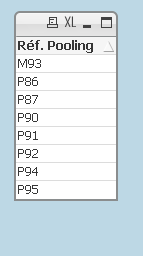# QlikView App Dev

Discussion Board for collaboration related to QlikView App Development.

Announcements
QlikWorld 2023, a live, in-person thrill ride. Save \$300 before February 6: REGISTER NOW!
cancel
Showing results for
Did you mean:Contributor II

## pivot table expression

Hi,

Basically I am working on a pivot table. Can anyone help me to solve the following equation? it would very much be appreciated.

 Number of reference « pooling Pxxx» : Number of [Ref. Pooling] starting with P***
 Number of reference « Mono Mxxx» : Number of [Ref. Pooling] starting with M*** or
Number of [Ref. Pooling] with [Code raison] « camion « mono-industriel »
 Pooling rate (camions théoriques) = Number of [Ref. Pooling] starting with P*** / total
Number of reference (« pooling Pxxx + Mono Mxxx »)Labels (2)

• ### Pivot Tables

1 Solution

Accepted SolutionsContributor III

Hi,

For number 1 and 2 can done by this dimension. and tick 'Suppress When Value is Null' in the same tab.

=IF(LEFT([Ref. Pooling],1)='M','MXXX',
IF(LEFT([Ref. Pooling],1)='P','PXXX'))

For number 3, go to Presentation tab, Tick 'Show Partial Sums'

For the expression, put COUNT(DISTINCT [Ref. Pooling])

Thanks.

3 RepliesContributor III

Hi, I'm not very understand about your requirement but I hope below helps.

=IF(LEFT([No Of Reference],1)='M','MXXX',
IF(LEFT([No Of Reference],1)='P','PXXX',
[No Of Reference]))

For Expression, put COUNT(DISTINCT [No Of Reference])

Thanks.

JeffContributor II
Author

Hi jefwork,

the following are the ones I need to be solved.

1.Number of [Ref. Pooling] starting with P***

2.Number of [Ref. Pooling] starting with M***

3.Number of [Ref. Pooling] starting with P*** / total
Number of reference (Number of [Ref. Pooling] starting with P*** + Number of [Ref. Pooling] starting with M***)Contributor III

Hi,

For number 1 and 2 can done by this dimension. and tick 'Suppress When Value is Null' in the same tab.

=IF(LEFT([Ref. Pooling],1)='M','MXXX',
IF(LEFT([Ref. Pooling],1)='P','PXXX'))

For number 3, go to Presentation tab, Tick 'Show Partial Sums'

For the expression, put COUNT(DISTINCT [Ref. Pooling])

Thanks.Community Browser